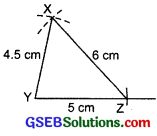# GSEB Solutions Class 7 Maths Chapter 10 Practical Geometry Ex 10.2

Gujarat Board GSEB Textbook Solutions Class 7 Maths Chapter 10 Practical Geometry Ex 10.2 Textbook Questions and Answers.

## Gujarat Board Textbook Solutions Class 7 Maths Chapter 10 Practical Geometry Ex 10.2Question 1.
Construct ∆XYZ in which XY = 4.5 cm, YZ = 5 cm and ZX = 6 cm.
Solution:
Steps of construction:
I. Draw a line segment YZ = 5 cm.
II. With centre Y and radius 4.5 cm, draw an arc.
III. With centre Z and radius 6 cm, draw another arc to cut the previous arc at X.
IV. Join YX and ZX.Thus, ∆XYZ is the required triangle.

Question 2.
Construct an equilateral triangle of side 5.5 cm.
Solution:
Steps of construction:
I. Draw a line segment BC = 5.5 cm.
II. With B as centre and radius 5.5 cm, draw an arc.
III. With centre C and radius 5.5 cm, draw another arc to cut the previous arc at A.
IV. Join BA and CA.Thus, ∆ABC is the required equilateral triangle.Question 3.
Draw ∆PQR with PQ = 4 cm, QR = 3.5 cm and PR = 4 cm. What type of triangle is this?
Solution:
Steps of construction:
I. Draw a line segment QR = 3.5 cm.
II. With Q as centre and radius 4 cm, draw an arc.
III. With R as centre and radius 4 cm, draw another arc such that it cuts the previous arc at R
IV. Join QP and RP.
Thus, ∆PQR is the required triangle.
Since, PQ = PR = 4 cmTherefore, PQR is an isosceles triangle.Question 4.
Construct ∆ABC such that AB = 2.5 cm, BC = 6 cm and AC = 6.5 cm. Measure ∠B.
Solution:
Steps of construction:
I. Draw a line segment BC = 6 cm.
II. With centre as B and radius 2.5 cm, draw an arc.
III. With centre C and radius 6.5 cm, draw another arc such that it cuts the previous arc at A.
IV. Join BA and CA.Thus, ∆ABC is the required triangle. On measuring, we find that ∠B = 90°.